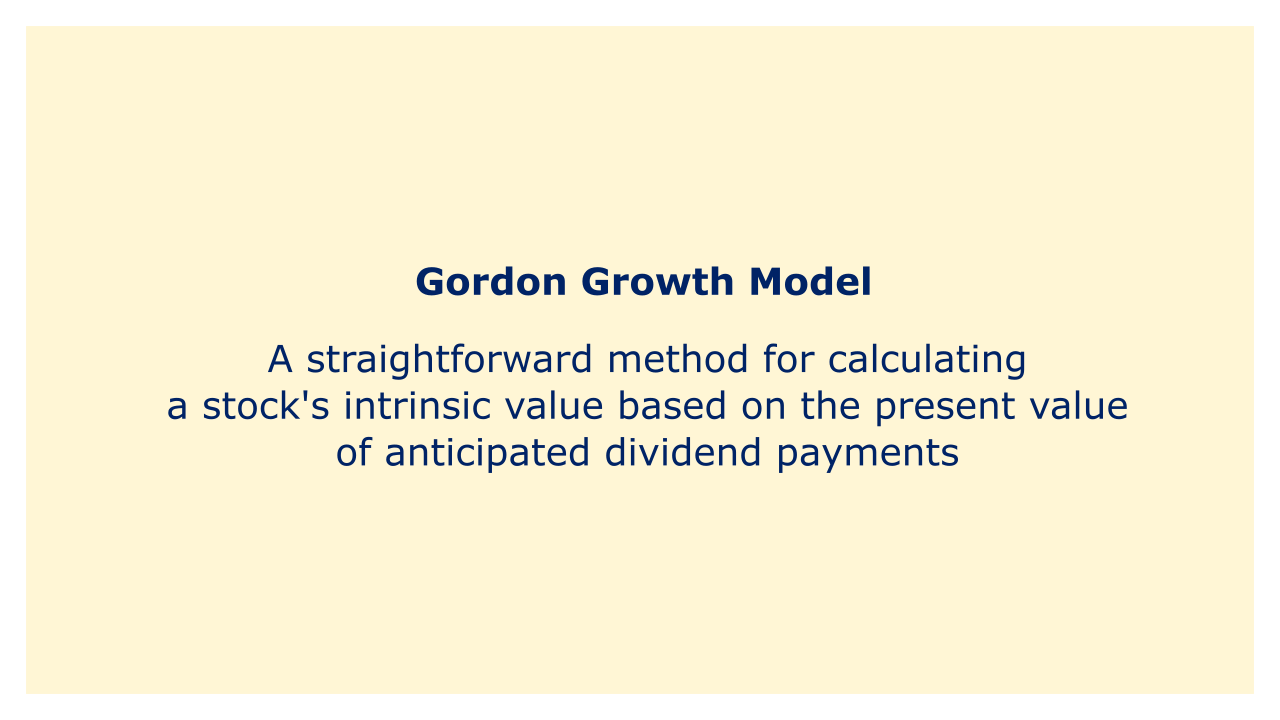# Gordon Growth ModelImage: Moneybestpal.com

### The Gordon Growth Model is a straightforward method for calculating a stock's intrinsic value based on the present value of anticipated dividend payments. It bears Myron Gordon's name because he introduced the model in 1959.

According to the Gordon Growth Model, a stock's value is equivalent to the present value of all upcoming dividend payments made by the company, discounted at a fixed rate. The dividend growth rate in the model is assumed to be constant over time. The "constant growth rate" or "g" is the name given to this growth rate.

The formula for the Gordon Growth Model is as follows:

P = D / (r - g)

Where r is the needed rate of return, g is the constant growth rate, P is the stock price, and D is the annual dividend payment.

Companies that pay dividends and have consistent, predictable growth rates can be valued using the Gordon Growth Model. It does, however, have some restrictions. The model, for instance, makes the unrealistic assumption that the growth rate is always constant. The model also largely depends on the growth rate assumption's correctness, which can be challenging to estimate over the long run.
Tags